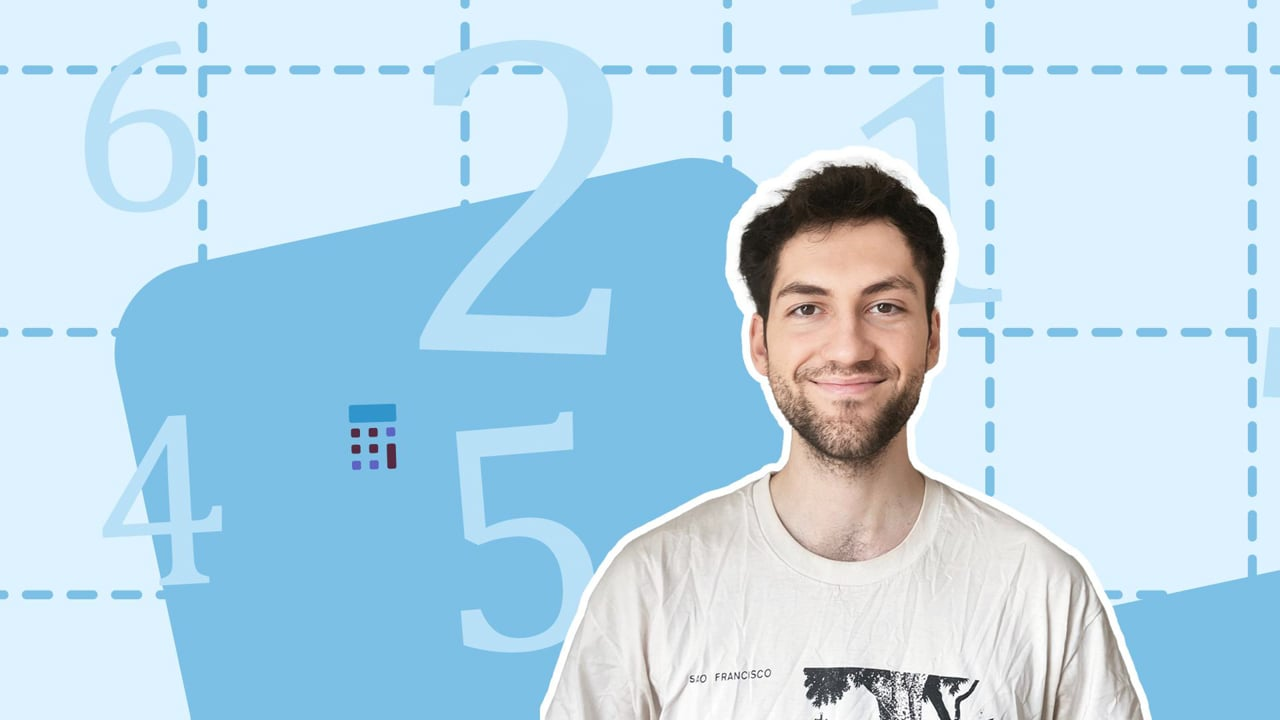Chapter overviewMaths

Number and place value

Multiplication and division

Fractions

Measurement

Geometry - properties of shapes

Geometry - position and direction

Statistics

Maths

# Multi-step problems with addition and subtraction0%

Summary

# Multi-step problems with addition and subtraction

## ​​In a nutshell

Addition and subtraction problems can involve multiple calculations. Knowing the order in which to do the calculations is essential.

With multi-step problems, any addition and subtraction methods you already know are applicable. You will have come across two methods: using mental arithmetic, perhaps involving number lines, and using the column method.

## Order of calculation

When adding only, the order in which you add the numbers doesn't matter.

When subtraction is involved, you should read the problem from left to right and calculate in that order.

Alternatively, recognise that subtracting a number is the same as adding the negative of that number.

##### Example 1

Since subtraction can be reframed as addition, the following expressions mean the same thing:

$5-2$​​

$5+(-2)$

$-2+5$​​

The point here is that when you subtract the number $x$, it is the same as adding the number $-x$. If you convert your subtractions into the addition of negative numbers, then the order doesn't matter.

##### Example 2

Rebecca has written one book, Sally has written three and Joe has also written three. Philip has written many more than these authors combined: Philip has written $44$ books. How many books have these four authors written combined?

You have four authors each with a number of books they wrote. For the combined value, you add these numbers up. Since you are adding the four numbers, the order doesn't matter. Start with $1+3$. This can be done mentally with a number line:

$\begin{array}{ccccccccccccc}&&&+1&&+1&&+1& \\& &&\curvearrowright &&\curvearrowright&&\curvearrowright \\0&&\underline1&&2&&3&&\underline4&&5 \\|&&|&&|&&|&&|&&| \\ \hline\end{array}$​​

So far you are up to $4$. Next add the other $3$:

$\begin{array}{ccccccccccccc}&&&+1&&+1&&+1& \\& &&\curvearrowright &&\curvearrowright&&\curvearrowright \\3&&\underline4&&5&&6&&\underline7&&8 \\|&&|&&|&&|&&|&&| \\ \hline\end{array}$​​

Now you are up to $7$ books. Finally add Philip's $44$ books. This can be done with the column method:

$\begin {array} {c c c}& \overset{1}{0}& 7 \\+ & 4 & 4 \\ \hline& 5 & 1 \\ \hline\end {array}$​​

Hence between these four authors, $\underline{51}$ books have been written.

##### Example 3

Katie is collecting stamps. She currently has $31$. She makes a deal with her friend, that she swaps $5$ of her stamps for $8$ of her friend's stamps. She then buys $14$ more stamps, before ditching $3$ because they are repeats. How many stamps does she have now in her collection?

The first step is to 'translate' the worded question into a mathematical statement. You therefore want to calculate:

$31-5+8+14-3$​​​

The $31$ is the initial number of stamps she has. The $-5$ accounts for the stamps she gives away in the swap and the $+8$ accounts for the stamps she gains in the swap. The $+14$ comes from the stamps she buys and the $-3$ comes from the stamps she gets rid of at the end.

Since there is addition and subtraction, you should calculate from left to right. Starting with the $31-5$​:

$\begin{array}{cccccccccccccc}&&&-1&&-1&&-1&&-1&&-1&&& \\& &&\curvearrowleft &&\curvearrowleft &&\curvearrowleft&&\curvearrowleft &&\curvearrowleft &&\\25&&\underline{26}&&27&&28&&29&&30&&\underline{31}&& \\|&&|&&|&&|&&|&&|&&| \\ \hline\end{array}$​​

Next add the $8$:

$\begin {array} {c c c}& \overset{1}{2}& 6 \\+ & & 8 \\ \hline& 3 & 4 \\ \hline\end {array}$​​

Now add the $14$:

$\begin {array} {c c c}& {3}& 4 \\+ & 1 & 4 \\ \hline& 4 & 8 \\ \hline\end {array}$​​

Finally, subtract the $3$:

$\begin{array}{cccccccccccccc}&&&-1&&-1&&-1&&& \\& &&\curvearrowleft &&\curvearrowleft &&\curvearrowleft&&\\44&&\underline{45}&&46&&47&&\underline{48}&&49 \\|&&|&&|&&|&&|&&| \\ \hline\end{array}$​​

So Katie has $\underline{45}$ stamps in her collection now.

Note: In this problem, both mental maths and the column method were used. This is ok - whichever method you decide is more suitable and preferable for the part of the problem is the best one.

## A shortcut

With some multi-step problems, the numbers involved can be manipulated to make the calculations easier.

##### Example 4

Calculate

$39+54+21$​​

Here you are adding all the numbers, so the order doesn't matter. But also notice that the $1$ from the $21$ would make the $39$ a whole number ($40$):

$39+54+21\\\space\\=39+54+20+1\\ \space\\=39+1+54+20\\\space\\=40+20+54\\\space\\=60+54$​​

So with a little rearranging, the multi-step problem has become a single addition.